Anúncio

# Notes and formulae mathematics

27 de Oct de 2014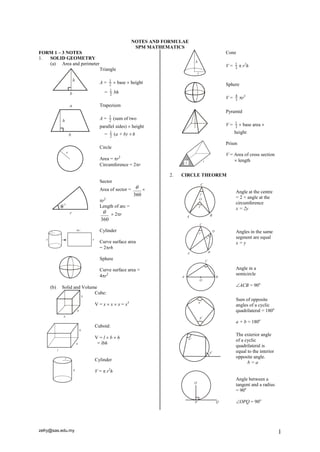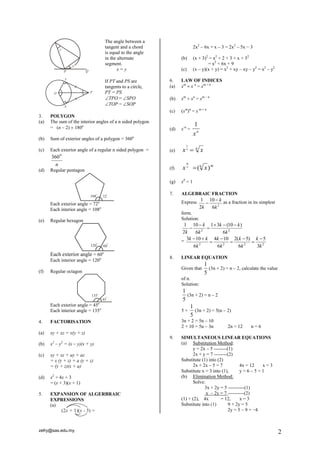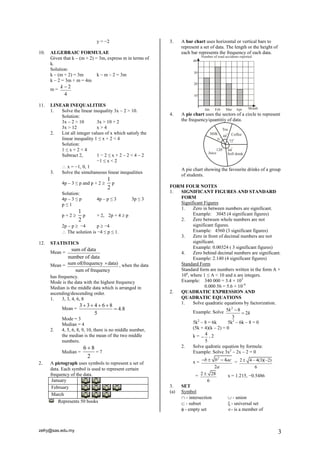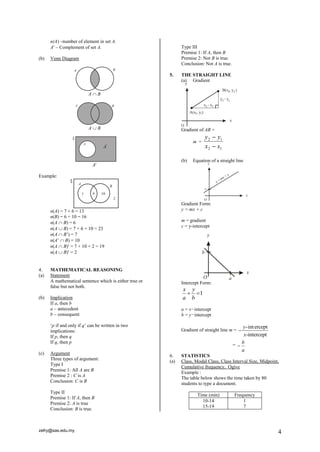Anúncio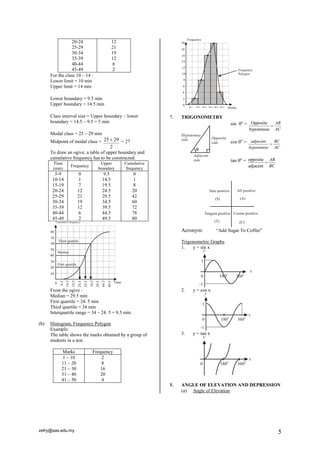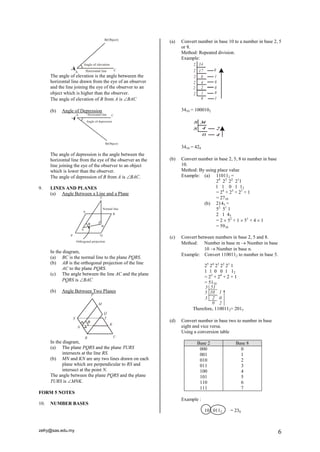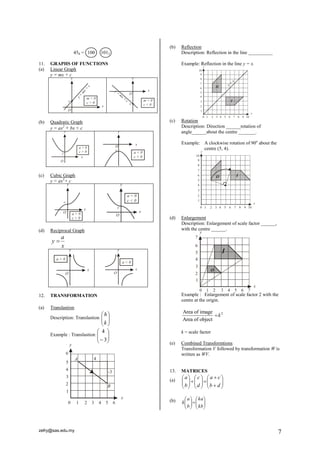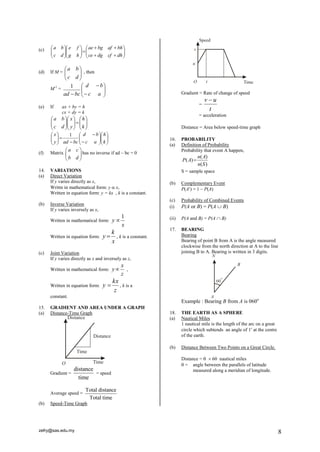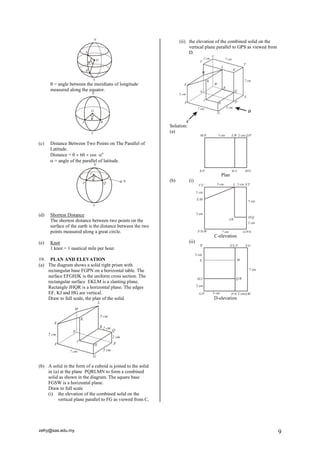Próximos SlideShares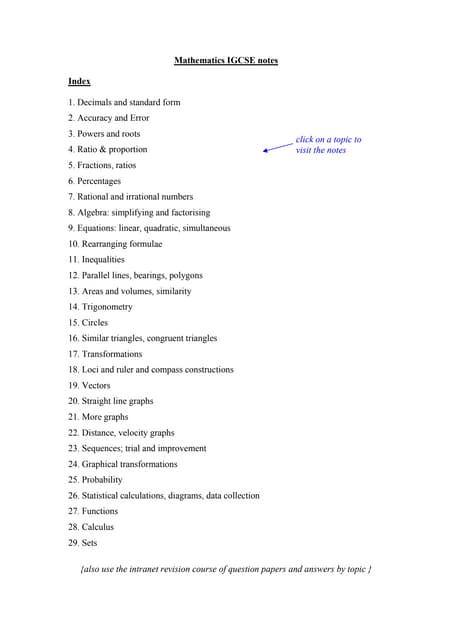Maths Revision Notes - IGCSE
Carregando em ... 3
1 de 9
Anúncio

### Notes and formulae mathematics

1. zefry@sas.edu.my 1 NOTES AND FORMULAE SPM MATHEMATICS FORM 1 – 3 NOTES 1. SOLID GEOMETRY (a) Area and perimeter Triangle A = 2 1  base  height = 2 1 bh Trapezium A = 2 1 (sum of two parallel sides)  height = 2 1 (a + b)  h Circle Area = r2 Circumference = 2r Sector Area of sector = 360   r2 Length of arc = 360   2r Cylinder Curve surface area = 2rh Sphere Curve surface area = 4r2 (b) Solid and Volume Cube: V = x  x  x = x3 Cuboid: V = l  b  h = lbh Cylinder V =  r2h Cone V = 3 1  r2h Sphere V = 3 4 r3 Pyramid V = 3 1  base area  height Prism V = Area of cross section  length 2. CIRCLE THEOREM Angle at the centre = 2 × angle at the circumference x = 2y Angles in the same segment are equal x = y Angle in a semicircle ACB = 90o Sum of opposite angles of a cyclic quadrilateral = 180o a + b = 180o The exterior angle of a cyclic quadrilateral is equal to the interior opposite angle. b = a Angle between a tangent and a radius = 90o OPQ = 90o
2. zefry@sas.edu.my 2 The angle between a tangent and a chord is equal to the angle in the alternate segment. x = y If PT and PS are tangents to a circle, PT = PS TPO = SPO TOP = SOP 3. POLYGON (a) The sum of the interior angles of a n sided polygon = (n – 2)  180o (b) Sum of exterior angles of a polygon = 360o (c) Each exterior angle of a regular n sided polygon = n 0 360 (d) Regular pentagon Each exterior angle = 72o Each interior angle = 108o (e) Regular hexagon Each exterior angle = 60o Each interior angle = 120o (f) Regular octagon Each exterior angle = 45o Each interior angle = 135o 4. FACTORISATION (a) xy + xz = x(y + z) (b) x2 – y2 = (x – y)(x + y) (c) xy + xz + ay + az = x (y + z) + a (y + z) = (y + z)(x + a) (d) x2 + 4x + 3 = (x + 3)(x + 1) 5. EXPANSION OF ALGERBRAIC EXPRESSIONS (a) 2x2 – 6x + x – 3 = 2x2 – 5x − 3 (b) (x + 3)2 = x2 + 2 × 3 × x + 32 = x2 + 6x + 9 (c) (x – y)(x + y) = x2 + xy – xy – y2 = x2 – y2 6. LAW OF INDICES (a) xm  x n = xm + n (b) xm  xn = xm – n (c) (xm)n = x m  n (d) x-n = n x 1 (e) x n  n x 1 (f) n m x n x m ( ) (g) x0 = 1 7. ALGEBRAIC FRACTION Express 2 1 10 2 6 k k k   as a fraction in its simplest form. Solution: 2 2 1 10 1 3 (10 ) 2 6 6 k k k k k k       = 2 2 2 2 3 10 4 10 2( 5) 5 6 6 6 3 k k k k k k k k k         8. LINEAR EQUATION Given that 1 5 (3n + 2) = n – 2, calculate the value of n. Solution: 1 5 (3n + 2) = n – 2 5 × 1 5 (3n + 2) = 5(n – 2) 3n + 2 = 5n – 10 2 + 10 = 5n – 3n 2n = 12 n = 6 9. SIMULTANEOUS LINEAR EQUATIONS (a) Substitution Method: y = 2x – 5 --------(1) 2x + y = 7 --------(2) Substitute (1) into (2) 2x + 2x – 5 = 7 4x = 12 x = 3 Substitute x = 3 into (1), y = 6 – 5 = 1 (b) Elimination Method: Solve: 3x + 2y = 5 ----------(1) x – 2y = 7 ----------(2) (1) + (2), 4x = 12, x = 3 Substitute into (1) 9 + 2y = 5 2y = 5 – 9 = −4
3. zefry@sas.edu.my 3 y = −2 10. ALGEBRAIC FORMULAE Given that k – (m + 2) = 3m, express m in terms of k. Solution: k – (m + 2) = 3m k – m – 2 = 3m k – 2 = 3m + m = 4m m = 2 4 k  11. LINEAR INEQUALITIES 1. Solve the linear inequality 3x – 2 > 10. Solution: 3x – 2 > 10 3x > 10 + 2 3x > 12 x > 4 2. List all integer values of x which satisfy the linear inequality 1 ≤ x + 2 < 4 Solution: 1 ≤ x + 2 < 4 Subtract 2, 1 − 2 ≤ x + 2 – 2 < 4 – 2 −1 ≤ x < 2  x = −1, 0, 1 3. Solve the simultaneous linear inequalities 4p – 3 ≤ p and p + 2  1 2 p Solution: 4p – 3 ≤ p 4p – p ≤ 3 3p ≤ 3 p ≤ 1 p + 2  1 2 p × 2, 2p + 4  p 2p – p  −4 p  −4  The solution is −4 ≤ p ≤ 1. 12. STATISTICS Mean = sum of data number of data Mean = sum of(frequency data) sum of frequency  , when the data has frequency. Mode is the data with the highest frequency Median is the middle data which is arranged in ascending/descending order. 1. 3, 3, 4, 6, 8 Mean = 3 3 4 6 8 4.8 5      Mode = 3 Median = 4 2. 4, 5, 6, 8, 9, 10, there is no middle number, the median is the mean of the two middle numbers. Median = 6 8 2  = 7 2. A pictograph uses symbols to represent a set of data. Each symbol is used to represent certain frequency of the data. January February March Represents 50 books 3. A bar chart uses horizontal or vertical bars to represent a set of data. The length or the height of each bar represents the frequency of each data. 4. A pie chart uses the sectors of a circle to represent the frequency/quantitiy of data. A pie chart showing the favourite drinks of a group of students. FORM FOUR NOTES 1. SIGNIFICANT FIGURES AND STANDARD FORM Significant Figures 1. Zero in between numbers are significant. Example: 3045 (4 significant figures) 2. Zero between whole numbers are not significant figures. Example: 4560 (3 significant figures) 3. Zero in front of decimal numbers are not significant. Example: 0.00324 ( 3 significant figures) 4. Zero behind decimal numbers are significant. Example: 2.140 (4 significant figures) Standard Form Standard form are numbers written in the form A × 10n, where 1 ≤ A < 10 and n are integers. Example: 340 000 = 3.4 × 105 0.000 56 = 5.6 × 10-4 2. QUADRATIC EXPRESSION AND QUADRATIC EQUATIONS 1. Solve quadratic equations by factorization. Example: Solve 2 5 8 2 3 k k   5k2 – 8 = 6k 5k2 – 6k – 8 = 0 (5k + 4)(k – 2) = 0 k = 4 5  , 2 2. Solve qudratic equation by formula: Example: Solve 3x2 – 2x – 2 = 0 x = 2 4 2 b b ac a    = 2 4 4(3)( 2) 6    = 2 28 6  x = 1.215, −0.5486 3. SET (a) Symbol  - intersection  - union  - subset  - universal set  - empty set - is a member of
4. zefry@sas.edu.my 4 n(A) –number of element in set A. A – Complement of set A. (b) Venn Diagram A  B A  B A Example: n(A) = 7 + 6 = 13 n(B) = 6 + 10 = 16 n(A  B) = 6 n(A  B) = 7 + 6 + 10 = 23 n(A  B‟) = 7 n(A‟  B) = 10 n(A  B) = 7 + 10 + 2 = 19 n(A  B) = 2 4. MATHEMATICAL REASONING (a) Statement A mathematical sentence which is either true or false but not both. (b) Implication If a, then b a – antecedent b – consequent „p if and only if q‟ can be written in two implications: If p, then q If q, then p (c) Argument Three types of argument: Type I Premise 1: All A are B Premise 2 : C is A Conclusion: C is B Type II Premise 1: If A, then B Premise 2: A is true Conclusion: B is true. Type III Premise 1: If A, then B Premise 2: Not B is true. Conclusion: Not A is true. 5. THE STRAIGHT LINE (a) Gradient Gradient of AB = m = 2 1 2 1 x x y y   (b) Equation of a straight line Gradient Form: y = mx + c m = gradient c = y-intercept Intercept Form:  1 b y a x a = x−intercept b = y−intercept Gradient of straight line m = -int ercept -intercept y x  = a b  6. STATISTICS (a) Class, Modal Class, Class Interval Size, Midpoint, Cumulative frequency, Ogive Example : The table below shows the time taken by 80 students to type a document. Time (min) Frequency 10-14 15-19 1 7
5. zefry@sas.edu.my 5 20-24 25-29 30-34 35-39 40-44 45-49 12 21 19 12 6 2 For the class 10 – 14 : Lower limit = 10 min Upper limit = 14 min Lower boundary = 9.5 min Upper boundary = 14.5 min Class interval size = Upper boundary – lower boundary = 14.5 – 9.5 = 5 min Modal class = 25 – 29 min Midpoint of modal class = 2 25  29 = 27 To draw an ogive, a table of upper boundary and cumulative frequency has to be constructed. Time (min) Frequency Upper boundary Cumulative frequency 5-9 10-14 15-19 20-24 25-29 30-34 35-39 40-44 45-49 0 1 7 12 21 19 12 6 2 9.5 14.5 19.5 24.5 29.5 34.5 39.5 44.5 49.5 0 1 8 20 42 60 72 78 80 From the ogive : Median = 29.5 min First quartile = 24. 5 min Third quartile = 34 min Interquartile range = 34 – 24. 5 = 9.5 min. (b) Histogram, Frequency Polygon Example: The table shows the marks obtained by a group of students in a test. Marks Frequency 1 – 10 11 – 20 21 – 30 31 – 40 41 – 50 2 8 16 20 4 7. TRIGONOMETRY sin o = Opposite hypotenuse AB AC  cos o = adjacent BC hypotenuse AC  tan o = opposite adjacent AB BC  Acronym: “Add Sugar To Coffee” Trigonometric Graphs 1. y = sin x 2. y = cos x 3. y = tan x 8. ANGLE OF ELEVATION AND DEPRESSION (a) Angle of Elevation
6. zefry@sas.edu.my 6 The angle of elevation is the angle betweeen the horizontal line drawn from the eye of an observer and the line joining the eye of the observer to an object which is higher than the observer. The angle of elevation of B from A is BAC (b) Angle of Depression The angle of depression is the angle between the horizontal line from the eye of the observer an the line joining the eye of the observer to an object which is lower than the observer. The angle of depression of B from A is BAC. 9. LINES AND PLANES (a) Angle Between a Line and a Plane In the diagram, (a) BC is the normal line to the plane PQRS. (b) AB is the orthogonal projection of the line AC to the plane PQRS. (c) The angle between the line AC and the plane PQRS is BAC (b) Angle Between Two Planes In the diagram, (a) The plane PQRS and the plane TURS intersects at the line RS. (b) MN and KN are any two lines drawn on each plane which are perpendicular to RS and intersect at the point N. The angle between the plane PQRS and the plane TURS is MNK. FORM 5 NOTES 10. NUMBER BASES (a) Convert number in base 10 to a number in base 2, 5 or 8. Method: Repeated division. Example: 2 2 2 2 2 2 34 17 8 4 2 1 0 0 1 0 0 0 1 3410 = 1000102 8 34 8 4 2 0 4 3410 = 428 (b) Convert number in base 2, 5, 8 to number in base 10. Method: By using place value Example: (a) 110112 = 24 23 22 211 1 1 0 1 12 = 24 + 23 + 21 + 1 = 2710 (b) 2145 = 52 51 1 2 1 45 = 2  52 + 1  51 + 4  1 = 5910 (c) Convert between numbers in base 2, 5 and 8. Method: Number in base m  Number in base 10  Number in base n. Example: Convert 1100112 to number in base 5. 25 24 23 22 21 1 1 1 0 0 1 12 = 25 + 24 + 2 + 1 = 5110 5 51 5 5 10 1 2 0 0 2 Therefore, 1100112= 2015 (d) Convert number in base two to number in base eight and vice versa. Using a conversion table Base 2 Base 8 000 001 010 011 100 101 110 111 0 1 2 3 4 5 6 7 Example : 10 0112 = 238
7. zefry@sas.edu.my 7 458 = 100 1012 11. GRAPHS OF FUNCTIONS (a) Linear Graph y = mx + c (b) Quadratic Graph y = ax2 + bx + c (c) Cubic Graph y = ax3+ c (d) Reciprocal Graph x a y  12. TRANSFORMATION (a) Translastion Description: Translastion         k h Example : Translastion          3 4 (b) Reflection Description: Reflection in the line __________ Example: Reflection in the line y = x. (c) Rotation Description: Direction ______rotation of angle______about the centre _______. Example: A clockwise rotation of 90o about the centre (5, 4). (d) Enlargement Description: Enlargement of scale factor ______, with the centre ______. Example : Enlargement of scale factor 2 with the centre at the origin. Area of image 2 Area of object k k = scale factor (e) Combined Transformtions Transformation V followed by transformation W is written as WV. 13. MATRICES (a)                             b d a c d c b a (b)                  kb ka b a k
8. zefry@sas.edu.my 8 (c)                              ce dg cf dh ae bg af bh g h e f c d a b (d) If M =         c d a b , then M-1 =            c a d b ad bc 1 (e) If ax + by = h cx + dy = k                          k h y x c d a b                             k h c a d b y ad bc x 1 (f) Matrix a c b d       has no inverse if ad – bc = 0 14. VARIATIONS (a) Direct Variation If y varies directly as x, Writtn in mathematical form: y  x, Written in equation form: y = kx , k is a constant. (b) Inverse Variation If y varies inversely as x, Written in mathematical form: 1 y x  Written in equation form: x k y  , k is a constant. (c) Joint Variation If y varies directly as x and inversely as z, Written in mathematical form: x y z  , Written in equation form: z kx y  , k is a constant. 15. GRADIENT AND AREA UNDER A GRAPH (a) Distance-Time Graph Gradient = distance time = speed Average speed = Total distance Total time (b) Speed-Time Graph Gradient = Rate of change of speed = t v  u = acceleration Distance = Area below speed-time graph 16. PROBABILITY (a) Definition of Probability Probability that event A happen, ( ) ( ) ( ) n A P A n S  S = sample space (b) Complementary Event P(A) = 1 – P(A) (c) Probability of Combined Events (i) P(A or B) = P(A  B) (ii) P(A and B) = P(A  B) 17. BEARING Bearing Bearing of point B from A is the angle measured clockwise from the north direction at A to the line joining B to A. Bearing is written in 3 digits. Example : Bearing B from A is 060o 18. THE EARTH AS A SPHERE (a) Nautical Miles 1 nautical mile is the length of the arc on a great circle which subtends an angle of 1 at the centre of the earth. (b) Distance Between Two Points on a Great Circle. Distance =   60 nautical miles  = angle between the parallels of latitude measured along a meridian of longitude.
9. zefry@sas.edu.my 9  = angle between the meridians of longitude measured along the equator. (c) Distance Between Two Points on The Parallel of Latitude. Distance =   60  cos o  = angle of the parallel of latitude. (d) Shortest Distance The shortest distance between two points on the surface of the earth is the distance between the two points measured along a great circle. (e) Knot 1 knot = 1 nautical mile per hour. 19. PLAN AND ELEVATION (a) The diagram shows a solid right prism with rectangular base FGPN on a horizontal table. The surface EFGHJK is the uniform cross section. The rectangular surface EKLM is a slanting plane. Rectangle JHQR is a horizontal plane. The edges EF, KJ and HG are vertical. Draw to full scale, the plan of the solid. (b) A solid in the form of a cuboid is joined to the solid in (a) at the plane PQRLMN to form a combined solid as shown in the diagram. The square base FGSW is a horizontal plane. Draw to full scale (i) the elevation of the combined solid on the vertical plane parallel to FG as viewed from C, (ii) the elevation of the combined solid on the vertical plane parallel to GPS as viewed from D. Solution: (a) Plan (b) (i) C-elevation (ii) D-elevation
Anúncio